Equilateral and Isoceles Triangle

Chapter 6 Class 7 Triangle and its Properties
Concept wise

### Find angle x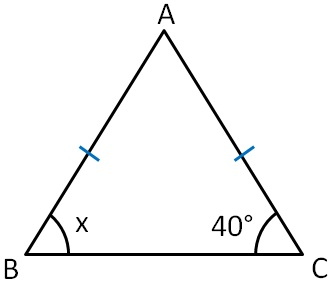In  ∆ABC,

AB = AC     (Given)

Therefore,

∠C = ∠B         (Angles opposite to equal sides are equal)

40° = x

x = 40°

### Find angle x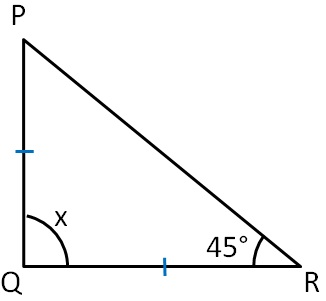In  ∆PQR,

PQ = QR          (Given)

Therefore,

∠R = ∠P           (Angles opposite to equal sides are equal)

45° = ∠P

∠P = 45°

Now, by Angle sum property,

∠P + ∠Q + ∠R = 180°

45° + x + 45° = 180°

x + 90° = 180°

x = 180° − 90°

x = 90 °

### Find angle x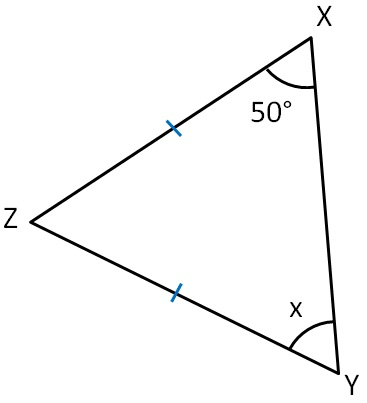In  ∆XYZ,

XZ = ZY         (Given)

Therefore,

∠Y = ∠X      (Angles opposite to equal sides are equal)

x = 50°

### Find angle x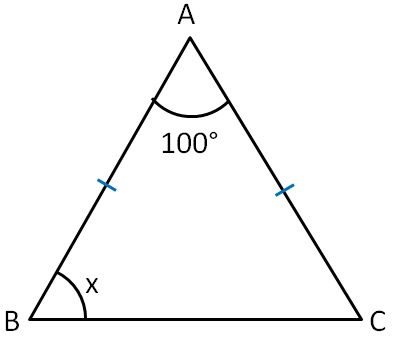In  ∆ABC,

AB = CA       (Given)

Therefore,

∠C = ∠B      (Angles opposite to equal sides are equal)

∠C  = x

Now, By angle sum property,

∠A + ∠B +∠C = 180°

100° + x + x = 180°

100° + 2x = 180°

2x = 180° − 100°

2x = 80°

x = 80° /2

x = 40°

### Find angle x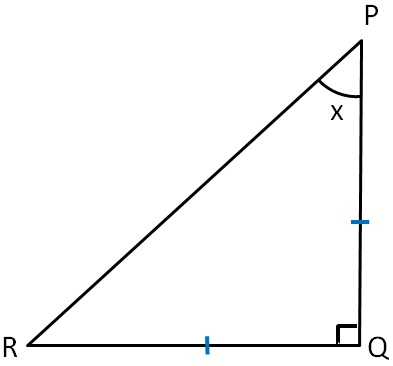In  ∆PQR,

QR = PQ      (Given)

Therefore,

∠P = ∠R    (Angles opposite to equal sides are equal)

x  = ∠R

∠R = x

Now, by angle sum property,

∠P + ∠Q + ∠R = 180°

x + 90° + x = 180°

90° + 2x = 180°

2x = 180° − 90°

2x = 90°

x = (90°)/2

x = 45°

### Find angle x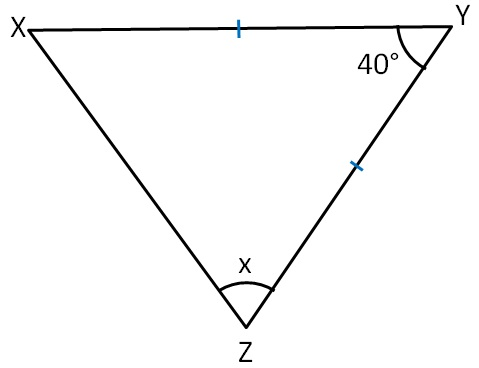In  ∆XYZ,

XY = YZ          (Given)

Therefore,

∠Z = ∠X         (Angles opposite to equal sides are equal)

x  = ∠X

∠X = x

Now, by angle sum property,

∠X + ∠Y + ∠Z = 180°

x + 40° + x = 180°

40° + 2x = 180°

2x = 180° − 40°

2x = 140°

x = (140°)/2

x = 70°

### Find angle x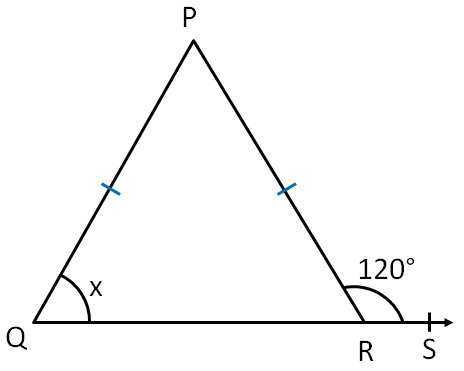In  ∆PQR,

PQ = PR      (Given)

Therefore,

∠PRQ = ∠Q       (Angles opposite to equal sides are equal)

∠PRQ = x

Now,

∠PRQ + ∠PRS = 180°      (Linear pair)

x + 120° = 180°

x = 180° − 120°

x = 60 °

### Find angle x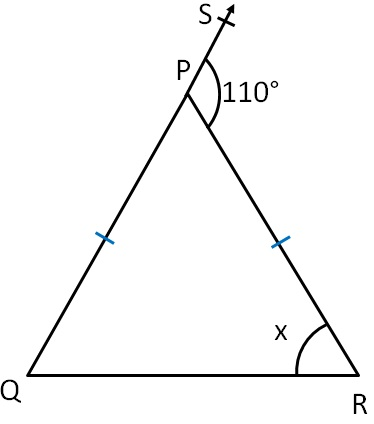In  ∆PQR,

PR = PQ       (Given)

Therefore,

∠Q = ∠R      (Angles opposite to equal sides are equal)

∠Q = x

We know that,

Exterior angle is equal to sum of interior opposite angles

∠SPR = ∠Q + ∠R

110° = x + x

110° = 2x

(110°)/2 = x

55° = x

x = 55°

### Find angle x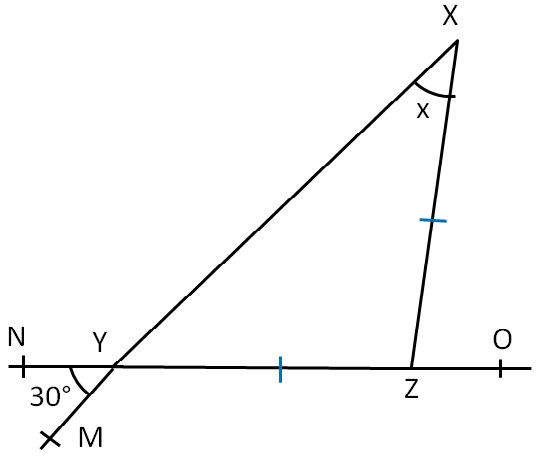Here

∠MYN  = ∠ZYX      (Vertically opposite angles)

30°  = ∠ZYX

∠ZYX = 30°

In ∆XYZ,

YZ = ZX           (Given)

Therefore,

30°  = ∠ZYX         (Angles opposite to equal sides are equal)

∠X = ∠ZYX

x = 30°

### Find angle x and y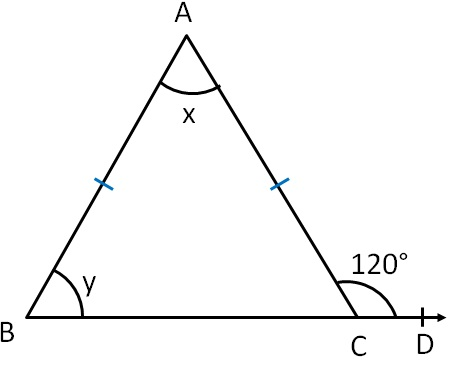In  ∆ABC,

AC = AB                  (Given)

Therefore,

∠B = ∠ACB           (Angles opposite to equal sides are equal)

y = ∠ACB

∠ACB = y

Now,

∠ACB + ∠ACD = 180°             (Linear pair)

y + 120° = 180°

y = 180° − 120°

y = 60°

We know that,

Exterior angle is equal to sum of interior opposite angles.

∠ACD = ∠A + ∠B

120 ° = x + y

120 ° = x + 60°

120 ° − 60° = x

60° = x

x = 60°

### Find angle x and y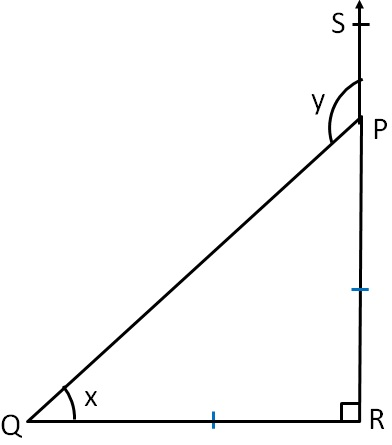In  ∆PQR,

QR = PR                        (Given)

Therefore,

∠QPR = ∠Q                (Angles opposite to equal sides are equal)

∠QPR = x

Now,

In Δ PQR

By Angle sum property

∠ QPR + ∠ Q + ∠ R = 180°

x + x + 90° = 180°

2x + 90° = 180°

2x = 180° – 90°

2x = 90°

x = (90°)/2

x = 45°

Also,

Exterior angle is sum of interior opposite angles

y = x + ∠ R

y = 45° + 90°

y = 135°

### Find angle x and y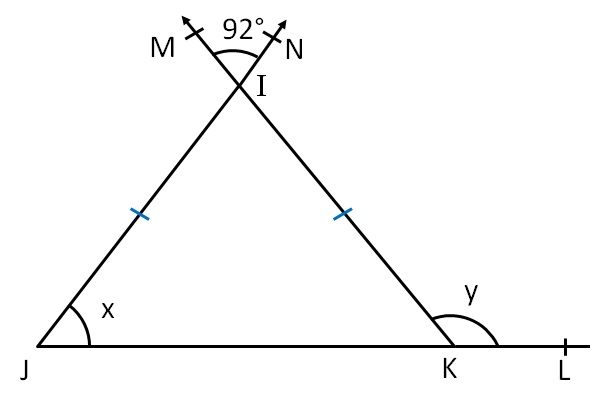Here,

∠JIK = ∠MIN        (Vertically opposite angle)

∠JIK = 92°

In ∆IJK,

IJ = IK                   (Given)

Therefore,

∠IKJ = ∠IJK        (Angles opposite to equal sides are equal)

∠IKJ = x

Now, By angle sum property,

∠IJK + ∠JKI + ∠KIJ = 180°

x + x + 92° = 180°

2x + 92° = 180°

2x = 180° − 92°

2x = 88°

x = (88°)/2

x = 44°

Hence,

IKJ = x

∠IKJ = 44°

Now,

∠IJK + ∠IKL = 180°       (Linear pair)

44° + y = 180°

y = 180° − 44°

y = 136°

x = 44° and y = 136°

Learn in your speed, with individual attention - Teachoo Maths 1-on-1 Class